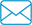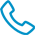800-871-5022

# Torque Conversion Chart Newton Meters to Inch Pounds

NewtonMetersInchPounds.pdf

 Newton Meters (N-m) Inch Pounds (in-lbs) Newton Meters (N-m) Inch Pounds (in-lbs) Newton Meters (N-m) Inch Pounds (in-lbs) Newton Meters (N-m) Inch Pounds (in-lbs) Newton Meters (N-m) Inch Pounds (in-lbs) 1 = 8.85 32 = 283.22 63 = 557.6 94 = 831.97 125 = 1106.34 2 = 17.7 33 = 292.07 64 = 566.45 95 = 840.82 126 = 1115.19 3 = 26.55 34 = 300.93 65 = 575.3 96 = 849.67 127 = 1124.04 4 = 35.4 35 = 309.78 66 = 584.15 97 = 858.52 128 = 1132.9 5 = 44.25 36 = 318.63 67 = 593 98 = 867.37 129 = 1141.75 6 = 53.1 37 = 327.48 68 = 601.85 99 = 876.22 130 = 1150.6 7 = 61.96 38 = 336.33 69 = 610.7 100 = 885.07 131 = 1159.45 8 = 70.81 39 = 345.18 70 = 619.55 101 = 893.93 132 = 1168.3 9 = 79.66 40 = 354.03 71 = 628.4 102 = 902.78 133 = 1177.15 10 = 88.51 41 = 362.88 72 = 637.25 103 = 911.63 134 = 1186 11 = 97.36 42 = 371.73 73 = 646.1 104 = 920.48 135 = 1194.85 12 = 106.21 43 = 380.58 74 = 654.96 105 = 929.33 136 = 1203.7 13 = 115.06 44 = 389.43 75 = 663.81 106 = 938.18 137 = 1212.55 14 = 123.91 45 = 398.28 76 = 672.66 107 = 947.03 138 = 1221.4 15 = 132.76 46 = 407.13 77 = 681.51 108 = 955.88 139 = 1230.25 16 = 141.61 47 = 415.99 78 = 690.36 109 = 964.73 140 = 1239.1 17 = 150.46 48 = 424.84 79 = 699.21 110 = 973.58 141 = 1247.96 18 = 159.31 49 = 433.69 80 = 708.06 111 = 982.43 142 = 1256.81 19 = 168.16 50 = 442.54 81 = 716.91 112 = 991.28 143 = 1265.66 20 = 177.01 51 = 451.39 82 = 725.76 113 = 1000.13 144 = 1274.51 21 = 185.87 52 = 460.24 83 = 734.61 114 = 1008.99 145 = 1283.36 22 = 194.72 53 = 469.09 84 = 743.46 115 = 1017.84 146 = 1292.21 23 = 203.57 54 = 477.94 85 = 752.31 116 = 1026.69 147 = 1301.06 24 = 212.42 55 = 486.79 86 = 761.16 117 = 1035.54 148 = 1309.91 25 = 221.27 56 = 495.64 87 = 770.01 118 = 1044.39 149 = 1318.76 26 = 230.12 57 = 504.49 88 = 778.87 119 = 1053.24 150 = 1327.61 27 = 238.97 58 = 513.34 89 = 787.72 120 = 1062.09 151 = 1336.46 28 = 247.82 59 = 522.19 90 = 796.57 121 = 1070.94 152 = 1345.31 29 = 256.67 60 = 531.04 91 = 805.42 122 = 1079.79 153 = 1354.16 30 = 265.52 61 = 539.9 92 = 814.27 123 = 1088.64 154 = 1363.01 31 = 274.37 62 = 548.75 93 = 823.12 124 = 1097.49 155 = 1371.87 Conversion Formula: Newton Meters x 8.85074579 = Inch Pounds